## Newton's Relations

Letbe the sum of the products of distinct Roots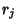of the Polynomial equation of degree(1)

where the roots are takenat a time (i.e.,is defined as the Elementary Symmetric Function)is defined for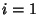, ...,. For example, the first few values ofare(2)(3)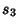(4)

and so on. Then(5)

This can be seen for a second Degree Polynomial by multiplying out,(6)

so(7)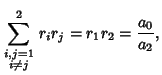(8)

and for a third Degree Polynomial,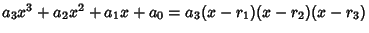(9)
so(10)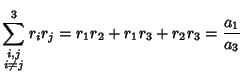(11)(12)